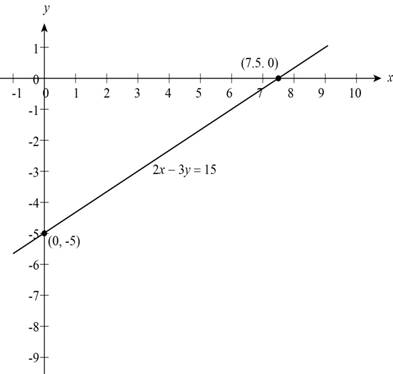# The slope-intercept form of linear equation 2 x − 3 y = 15 , draw its graph and the of slope and y -intercept of line.### Precalculus: Mathematics for Calcu...

6th Edition
Stewart + 5 others
Publisher: Cengage Learning
ISBN: 9780840068071### Precalculus: Mathematics for Calcu...

6th Edition
Stewart + 5 others
Publisher: Cengage Learning
ISBN: 9780840068071

#### Solutions

Chapter 1, Problem 19T
To determine

## The slope-intercept form of linear equation 2x−3y=15 , draw its graph and the of slope and y-intercept of line.

Expert Solution

The slope-intercept form of given linear equation is y=23x5 , the slope of line is 23 and the y-intercept of line is 5 .

### Explanation of Solution

Given:

The linear equation is 2x3y=15 .

Calculation:

Section1:

The slope-intercept form is y=23x5 .

The slope-intercept form of line is,

y=mx+b

Where,

m is slope of line.

b is y-intercept of line.

Rewrite the given equation in x-term for y to get the equation,

2x3y=153y=2x+15y=23x+153y=23x5

Thus, the slope-intercept form is y=23x5 .

Section2:

The given linear equation is 2x3y=15 .

The equation is linear so its graph is a line and there should be two pointsto draw a line.

Substitute 0 for y to get x-intercept,

2x3(0)=15x=152x=7.5

Substitute 0 for x to get y-intercept,

2(0)3y=153y=15y=153y=5

Two points (7.5,0) and (0,5) are obtained from x- and y-intercepts and through these two points the graph of linear equation is shown in Figure (1).

Figure (1) shows the graph of line 2x3y=15 passes through points (7.5,0) and (0,5) .Figure (1)

Section3:

The formula of slope-intercept form of line is,

y=mx+b

Where,

• m is slope of line.
• b is y-intercept of line.

The slope-intercept form of given linear equation from section1 is,

y=23x5

Compare the above equation with formula get the value of slope m=23 and the value of y-intercept b=5 .

Thus, the slope of line is 23 and y-intercept of line is 5 .

### Have a homework question?

Subscribe to bartleby learn! Ask subject matter experts 30 homework questions each month. Plus, you’ll have access to millions of step-by-step textbook answers!